Mobile QR Code1. (Department of Electronic Engineering, Chonnam National University / Gwangju, 61186, Korea xiaomeng199373@gmail.com, sichoi@jnu.ac.kr )

PointNet, PointSIFT, Point cloud, Semantic segmentation

## 1. Introduction

A 3D point cloud is a set of data points in space. Point clouds are generally produced by 3D scanners that measure many points on external surfaces of objects around them. 3D point clouds are often used as input for computer vision. 3D point cloud perception usually includes three major tasks: 3D object classification, 3D object detection, and 3D semantic segmentation. Among these, the semantic segmentation of 3D point clouds is the most challenging.

In computer vision, semantic segmentation is done to segment images or point clouds to distinguish between different meaningful segments. Semantic segmentation divides an image or point cloud into semantically meaningful parts and then categorizes each part into a predefined class. Identifying objects within different point clouds or image data is very useful in many applications. However, there are many challenges in 3D semantic segmentation. The sparseness of point clouds makes most training algorithms inefficient, while the relationship between points is not obvious and is difficult to represent.

In previous years, in order to solve these problems, many methods have been proposed to manually create functional representations of point clouds that were adjusted for 3D object detection, such as a 3D CNN  and polygon meshes [2,3]. A 3D CNN is based on a 2D CNN and convolves the 3D mesh after the point cloud has been voxelized. The goal is to learn the features of the point cloud and perform classification and segmentation operations. However, these manual designs can lead to information bottlenecks, which prevent such methods from effectively utilizing the three-dimensional shape information fully. It also increases the amount of calculation required, which results in reduced computational efficiency.

Recently, the PointNet architecture  was proposed. It runs directly on point clouds instead of on 3D voxels or grids. It not only speeds up calculation, but also significantly improves segmentation performance. PointNet is an end-to-end deep neural network that learns point-wise features directly from point clouds. In this study, we designed a point cloud semantic segmentation algorithm based on PointNet, in which the PointSIFT  module is applied.

This paper is organized as follows. In section 2, we introduce the PointNet algorithm and the PointSIFT module along with our algorithm architecture. Section 3 shows the results of our experiments on semantic segmentation of point clouds. Finally, conclusions are covered in section 4.

## 2. Point Cloud Semantic Segmentation

### 2.1 PointNet Architecture

Qi et al.  designed a deep learning framework named PointNet, which uses unordered points directly as input. The PointNet architecture is shown in Fig. 1. PointNet basically has three main components: a local feature extraction layer, a symmetric function for summarizing information from all local features, and a global feature extraction layer for aligning global features for various learning tasks. The point cloud is represented by a set of 3D points $\left\{P_{i}|i=1,\cdots ,n\right\}$, where each point $P_{i}$ is a vector containing $\left(x,\,\,y,\,\,z\right)$ coordinates plus an additional feature channel.

A multilayer perceptron (MLP) extends the original 3D points in the point cloud to very high dimensions. In this way, a local feature of a 3D point can be output. The characteristics of each point are shared in the PointNet model. Based on this sharing, the features of the high-dimensional points become different. Considering all the local features of the point cloud, the values of one dimension are integrated into a set of PointNet local eigenvalues in a local feature dimension. Thus, PointNet can use a symmetric function to select a representative value for each set as an output that includes global features of the point cloud.

This step is implemented using an $n\times 1$ max-pooling operator, where $\textit{n}$ is the number of points in the observation point cloud, and the representative value is the maximum of each individual dimension value set . This technique solves the problem of out-of-order points being represented by point clouds. After the global features are extracted, these global features are used by the MLP to achieve different goals, such as object classification and semantic segmentation.

### 2.2 PointSIFT Module

The PointSIFT module is implemented based on a SIFT algorithm and involves two key attributes: orientation-encoding and scale-awareness. Fig. 2 shows the architecture of the PointSIFT module. Orientation-encoding (OE) convolution is the basic unit in the PointSIFT block that captures surrounding points. Fig. 3 shows the OE unit of the PointSIFT module. Given a point $p_{0}$, its corresponding feature is represented by $f_{0}$. The 3D space with $p_{0}$ as the center point can be divided into 8 subspaces (octants) in 8 directions.

From the $\textit{k}$ nearest neighbors of $p_{0}$, if there is no point in the search radius $\textit{r}$ within a certain octant, the feature of the subspace is considered to be equal to $f_{0}$. Assuming that the input point cloud is $n\times d$, after the step is over, each feature has information in eight directions around it, which becomes $n\times 8\times d$. For the convolution operation to sense the direction information, a three-phase convolution is performed on the $\textit{X}$, $\textit{Y}$, and $\textit{Z}$ axes. For feature encoding of the searched $\textit{k}$-nearest neighbor points, $M\in R^{2\times 2\times 2\times d}$, the first three dimensions represent the coding of the points on the eight subspaces. The three-phase convolution is expressed as:

##### (1)
$$M_{1}=g\left[Conv\left(A_{x},M\right)\right]\in R^{2\times 2\times d},$$ $$M_{2}=g\left[Conv\left(A_{y},M\right)\right]\in R^{2\times d},$$ $M_{3}=g\left[Conv\left(A_{z},M\right)\right]\in R^{1\times d},$

where $A_{x},A_{y},\,\,\mathrm{and}\,\,A_{z}$ are the convolution weights to be optimized; $Conv_{x}$, $Conv_{y}$, and $Conv_{z}$ represent the respective convolution operations on the $\textit{X}$, $\textit{Y}$, and $\textit{Z}$ axes; and $g\left(x\right)$ represents $ReLU\left(\textit{BatchNorm}\left(\cdot \right)\right)$.

The scale-awareness of the PointSIFT module is formed by stacking direction coding units. A higher-level OE unit has a larger receptive field than a lower-level OE unit. By constructing a hierarchy of OE units, we obtain a multi-scale representation of local regions in a point cloud. For a certain direction coding convolution unit, the features in the 8 direction fields are extracted, the receptive field can be regarded as the $\textit{k}$ field in 8 directions, and each field corresponds to one feature point.

The features of the various scales are connected by several identification shortcuts and transformed by a point-by-point convolution of another output $\textit{d}$-dimensional multi-scale feature. In the process of joint optimization feature extraction and point-by-point convolution of integrated multi-scale features, the neural network learns to choose or adhere to appropriate scales, which makes our network-scale software possible. Ideally, we stack $\textit{i}$ times, and the receptive field is $8^{i}$ points. Finally, these layers are spliced together through a shortcut followed by pointwise convolution ($1\times 1$ convolution) so that the network can choose the appropriate scale with training.

##### Fig. 2. Architecture of PointSIFT module.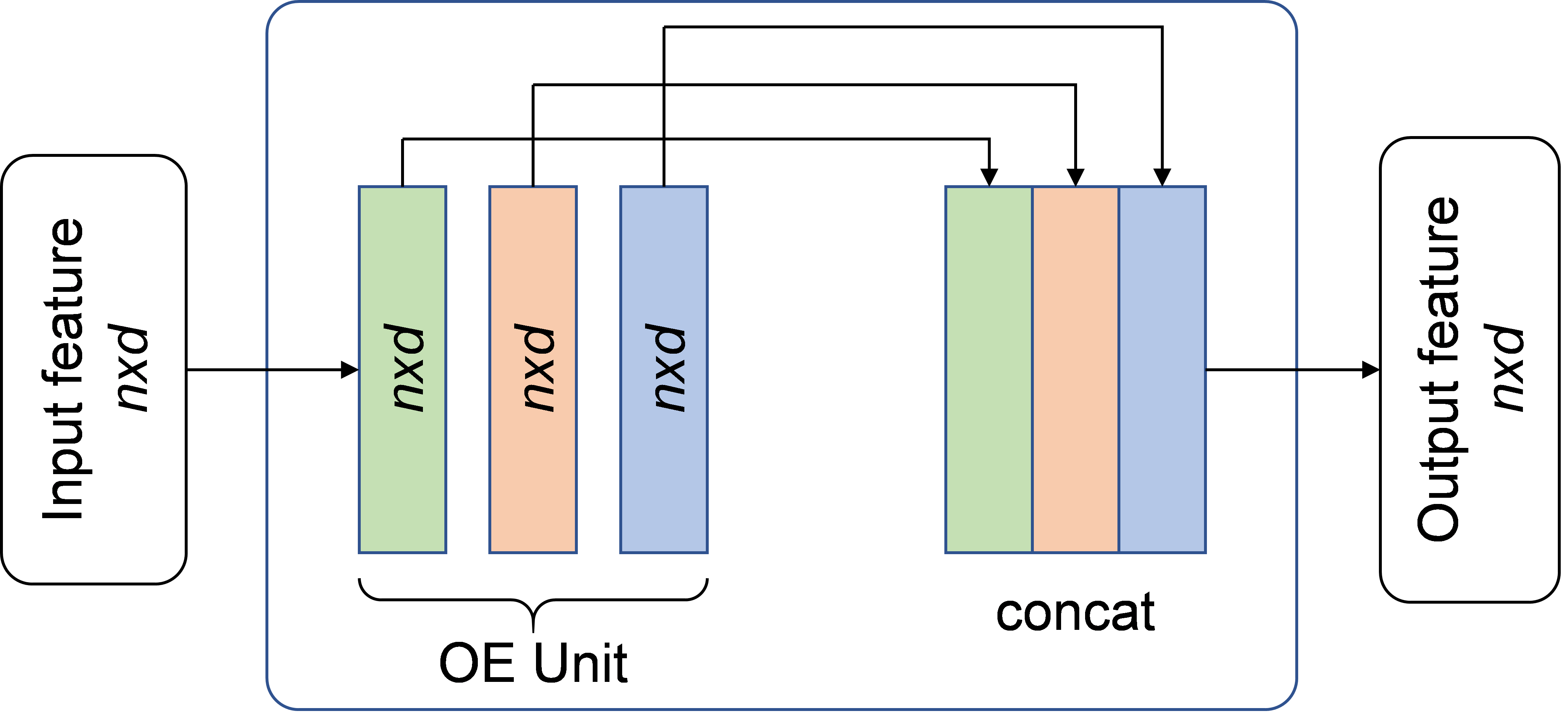##### Fig. 3. Orientation-Encoding unit (a) Point cloud in 3D space, (b) Nearest neighbor search in eight octants.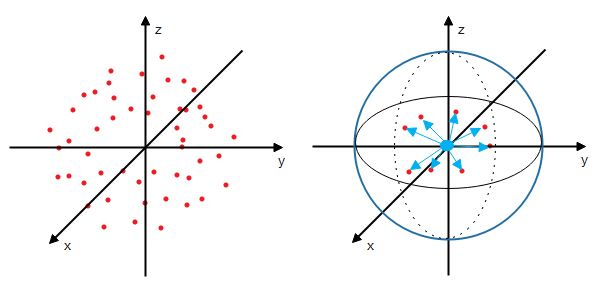### 2.3 S-PointNet Architecture

We designed a new semantic segmentation algorithm for point clouds named S-PointNet, which is based on the PointNet architecture. The PointSIFT module is integrated into the PointNet architecture to improve the representation ability. Fig. 4 shows the S-PointNet architecture. The PointSIFT module is applied to extract the local features. By combining the local features and the global features, we can extract new features for each point and perform semantic segmentation.

The proposed deep learning framework S-PointNet directly uses disordered point clouds as inputs. The input to the algorithm is $\textit{n}$ points with dimension $\textit{d}$ (e.g., $\textit{x, y, z,}$ color parameters, and normal vectors). Each input point is first extended to a vector with 64-dimensional features using the MLP. Then it is connected to the PointSIFT module to perform two 64-dimensional transformations to learn the local orientation of each point and output it.

The entire point cloud is then expanded to 1024 dimensions using 3 dimensionally expanded MLPs, which is sufficient to preserve almost all the point cloud information. The output vector matrix is symmetrically max-pooling and takes on global features. The vector operated by max-pooling retains the global feature and loses the feature of a single point, so we use the reshape function to map the vector with global features to all points and join it to the previously obtained local feature vector. Thus, each point can search among the global features and find the category to which it belongs.

The vector is then reduced to 128 dimensions using 3 MLPs of progressively lower dimensionality. Finally, we output data for $\textit{m}$ categories using the full join layer, where \textit{m} is the number of object categories for all points. We also add $\textit{ReLU}$ and $\textit{BatchNorm}$ functions to all MLPs and the full join layer to reduce overfitting.

##### Fig. 4. S-PointNet architecture. MLP stands for multilayer perceptron, and numbers in bracket are layer sizes.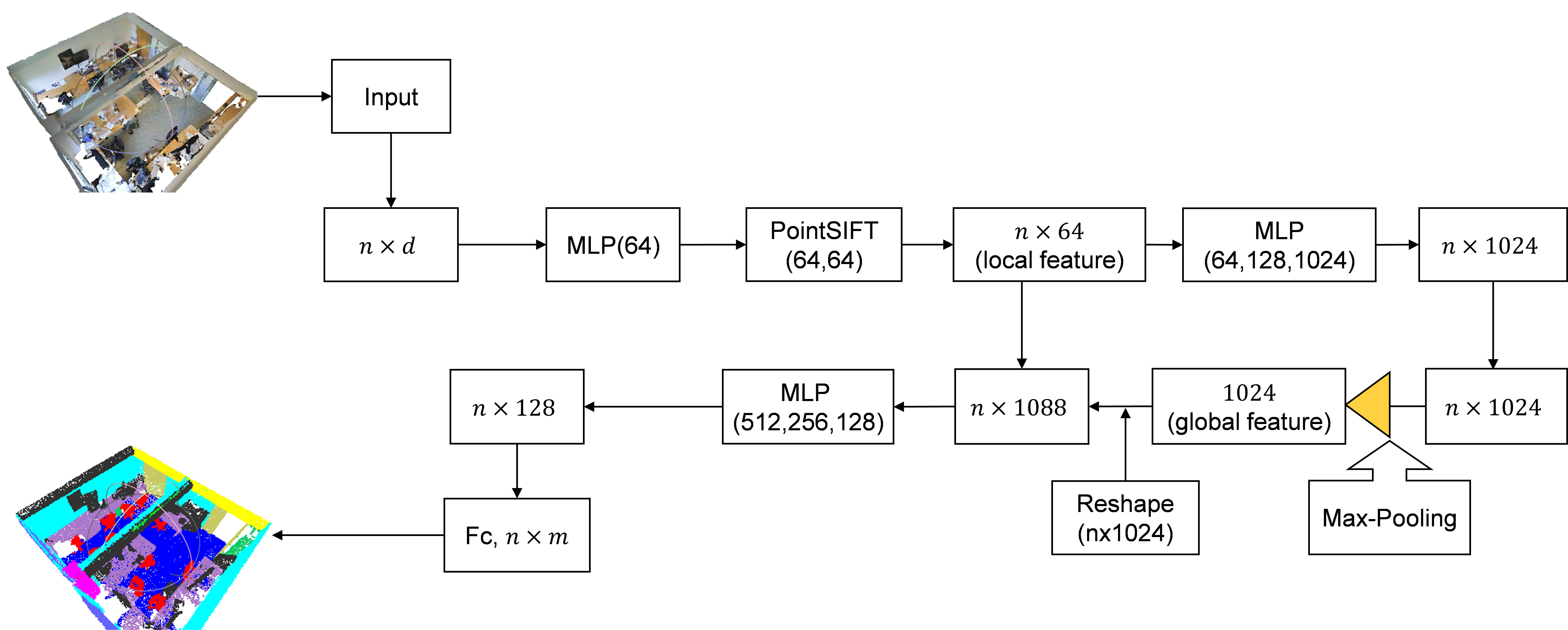## 3. Performance Evaluation

We conducted experiments using the Stanford 3D semantic parsing dataset . The dataset contains 3D scans from Matterport scanners of 6 distinct areas including 271 rooms. Each point in the scan is annotated with one semantic tag from 13 possible categories (chairs, tables, floors, and walls, among others, as well as a clutter tag). We divide the room into 1-m $\times$ 1-m $\times$ 1-m blocks in the training data, and each point is represented by a 9-dimensional vector including XYZ, RGB, and spatial normalized position (0 - 1) data. 4096 points are randomly selected in each block during training.

We follow the same protocol as a previous study  and use the $\textit{k}$-fold strategy for training and testing. Before carrying out the segmentation prediction, we used a loss of 0.7 on the fully connected layer. The decay rate of $\textit{BatchNorm}$ was gradually increased from 0.5 to 0.99. We used the Adam optimizer with an initial learning rate of 0.001, a momentum of 0.9, and a batch size of 24. The platform used had an Intel i9-9900K with an NVIDIA GTX2080Ti GPU.

Table 1 shows the semantic segmentation results of each algorithm when using the S3DIS dataset. Compared with other methods, S-PointNet achieves better performance than PointNet and 3D CNN. For the evaluation metrics, we used the mean class-wise intersection over union (mIoU), the mean class-wise accuracy (mAcc), the overall point-wise accuracy (OA), and the Dice similarity coefficient (DSC). The scores of each algorithm are shown in Table 2. MIoU is the intersection of the prediction area and the actual area divided by the union of the prediction area and the actual area. DSC is a set similarity measurement functions that are usually used to calculate the similarity of two samples. When compared with PointNet and 3D CNN, our algorithm shows better performance, but PointCNN  shows the best performance.

Table 3 shows the parameter numbers, FLOPs, and running time of each algorithm. FLOP stands for floating-point operation, and M'' stands for million. An NVIDIA GTX 2080Ti GPU was used for the experiment with 2048 input points and a batch size of 24. The S-PointNet algorithm has 4.0M parameters, 490M FLOPs for training, 161M FLOPs for inference, 0.43 sec per batch for training, and 0.11 sec per batch for inference. Even though PointCNN shows better performance than S-PointNet, our algorithm outperforms other methods in training time and inference efficiency.

Fig. 5 shows the visualization results of semantic segmentation of the PointNet and S-PointNet architectures. Fig. 5(a) shows the original raw point cloud data for three different spaces in the same dataset. Fig. 5(b) shows the ground-truth for three different spaces. Fig. 5(c) shows the semantic segmentation results of PointNet. Fig. 5(d) shows the semantic segmentation results of the proposed algorithm. The semantic segmentation results show that the point clouds are correctly classified and categorized as tables, chairs, walls, etc. The overall segmentation results show that the performance of S-PointNet is satisfactory.

##### Table 1. Semantic segmentation results on S3DIS dataset with 6-folds cross validation.
 Method ceiling floor wall beam column window door table chair sofa bookcase board clutter PointNet 88.41 88.15 68.83 39.62 20.22 49.61 51.68 55.04 42.88 7.72 38.61 31.83 35.48 3D CNN 90.17 96.48 70.16 0.00 11.40 33.36 21.12 70.07 76.12 37.46 57.89 11.16 41.61 PointCNN 93.40 97.13 81.48 54.58 40.30 66.64 54.63 71.82 63.18 35.61 62.22 58.84 58.50 S-PointNet 88.87 92.35 70.08 40.29 29.43 51.21 53.84 56.54 45.76 16.52 40.91 38.13 37.45
##### Table 2. Comparison of OA, mAcc, mIoU, and DSC.
 Method OA mAcc mIoU DSC PointNet 78.23 65.50 47.55 31.19 3D CNN 77.59 54.91 47.46 31.11 PointCNN 87.36 75.61 64.49 47.59 S-PointNet 80.10 68.03 50.88 34.12
##### Table 3. Parameter number, FLOPs, and running time.
 Method PointNet  3D CNN  PointCNN  S-PointNet Parameters 3.5M 30.7M 4.4M 4.0M FLOPs Training 440M 872M 930M 490M Inference 147M 420M 253M 161M Time Training 0.68 s 9.53 s 0.61 s 0.43 s Inference 0.15 s 5.50 s 0.25 s 0.11 s
##### Fig. 5. Semantic segmentation results of S-PointNet (a) Raw point cloud data, (b) ground-truth, (c) results of PointNet, (d) results of proposed S-PointNet algorithm.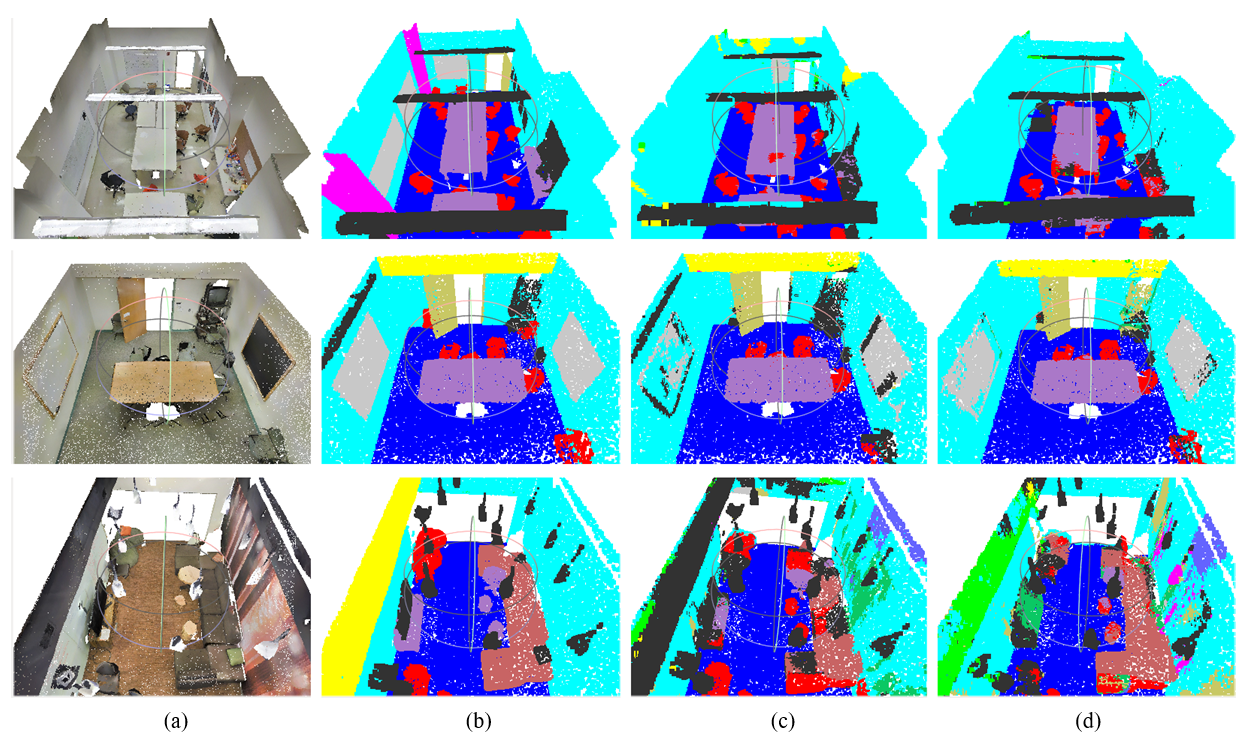## 4. Conclusion

In this study, we designed a new semantic segmentation algorithm for 3D point clouds based on the PointNet architecture. The proposed S-PointNet algorithm was superior to other methods for semantic segmentation using a standard dataset, including the original PointNet. An MLP was used to extend the local features of a 3D point cloud to high-dimensional space, and the scale of the scanned data was considered and processed by the PointSIFT module. Finally, the local features were connected with the global features, and the semantic segmentation results were output. We also carried out experiments that showed the enhanced effectiveness of the proposed algorithm.

### ACKNOWLEDGMENTS

This research was supported by Basic Science Research Program through the National Research Foundation of Korea(NRF) funded by the Ministry of Education(NRF-2018R1D1A1B07048868).

### REFERENCES

1
Tchapmi L. P., Choy C. B., Armeni I., Gwak J., Oct. 2017, SEGCloud: Semantic Segmentation of 3D Point Clouds, in Proc. of 3D Vision (3DV) 2017, Vol. , No. , pp. 537-5472
Bruna J., Zaremba W., Szlam A., Lecun Y., Apr. 2014, Spectral Networks and Locally Connected Networks on Graphs, in Proc. of ICLR 2014, Vol. , No. , pp. 1-14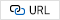3
Masci J., Boscaini D., Bronstein M. M., Vandergheynst P., Dec. 2015, Geodesic Convolutional Neural Networks on Riemannian Manifolds, in proc. of ICCVW 2015, pp. 832-8404
Charles R. Q., Su H., Kaichun M., Guibas L. J., Jul. 2017, PointNet: Deep Learning on Point Sets for 3D Classification and Segmentation, in proc. of CVPR 2017, pp. 77-855
Jiang M., Wu Y., Zhao T., Zhao Z., Lu C., Jul. 2018, PointSIFT: A SIFT-like Network Module for 3D Point Cloud Semantic Segmentation, ArXiv abs/1807.00652, pp. 1-106
Armeni I., Sener O., Zamir A. R., Jiang H., Brilakis I., Fischer M., Savarese S., Jun. 2016, 3D Semantic Parsing of Large-Scale Indoor Spaces, in proc. of CVPR 2016, pp. 1534-15437
Li Y., Bu R., Sun M., Wu W., Di X., Chen B., Nov. 2018, PointCNN: Convolution on X-Transformed Points, in proc. of NIPS 2018, pp. 820-830## Author

Jiongyi Meng received his Bachelor of Computer Engineering from Chonnam National University (JNU) in South Korea in 2017. In February 2020, he went on to obtain a master's degree from Chonnam University. He is currently a PhD student in Electronic Engineering at Chonnam National University. He continues to conduct research on 3D point cloud object detection and classification, and participates in the development of the S-PointNet algorithm. His current research interests include 3D point cloud segmentation, 2D image and 3D point cloud fusion object detection.

Su-il Choi received his B.S. degree in electronics engineering from Chonnam National University, South Korea, in 1990, and his M.S. and Ph.D. degrees from the Korea Advanced Institute of Science and Technology (KAIST), South Korea, in 1992 and 1999, respectively. From 1999 to 2004, he was with the Network Laboratory in ETRI. Since 2004, he has been with the faculty of Chonnam National University, where he is currently a Professor with the Department of Electronic Engineering. His research interests are in optical communications, access networks, QoS, and LiDAR based object detection and segmentation.